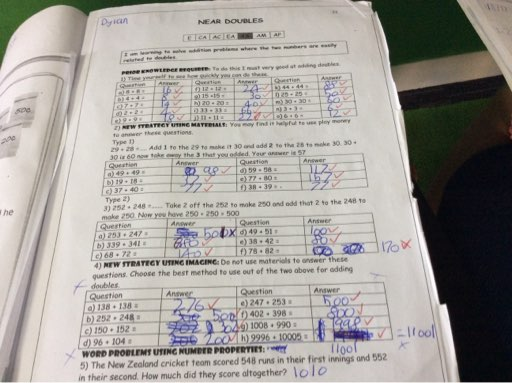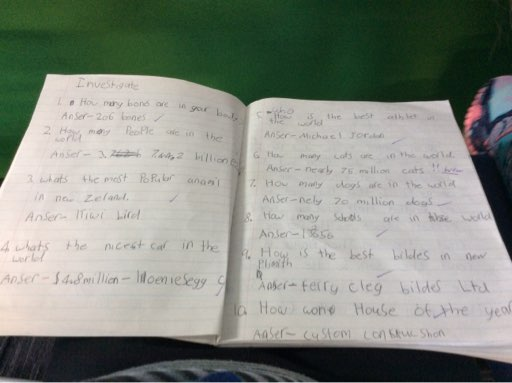## Monday, 6 November 2017

### MathsMaths 8+8 = 16 1008+990= 1998 252+248= 500 78+82= 170 37+40= 77 247+253= 500 49+51= 100 11+11= 22 38+39= 77 77+80= 157

### Investigate SkillsInvestigate skills 1. How many bones are in your body. Answer: 206 bones. 2. How many people are in the world 🌎 Answer: 7.442 people 3.what is the most popular animal in New Zealand 🇳🇿 Answer: kiwi bird 🐦 4. What is the nicest car 🚗 in the world Answer: koeniesegg ccxr 5. How many dogs are in the world Answer: 70 million dogs